Power Dividers and Combiners

Copy from the web page: http://www.bibel-reflektion.de/html/circuits_and_components.html

Power dividing and combining is a standard requirement  for RF- Designers. The following circuits or components are used :

• The Wheatstone Bridge coupler.
• Wave line and twisted wire wave line combiners and dividers.
• Line Hybrids.
• Ring and Brunch Hybrids.
• Wilkinson Dividers.

1.0 The Wheatstone Bridge as a coupler.

The easiest way to couple a signal in the range from DC to 500 MHz  into an other path, is the use of a Wheatstone bridge. The advantage of this circuit is: He is only resistive, has no transformer no complex wiring and is ideal for digital broadband application. But the outputs have no reference to ground. Fig.1 Therefore this bridge is seldom used. At lower frequencies , the 2 outputs can drive a RF-Operational Amplifier . Fig1 shows a circuit and its resistance values depending on the coupling factor a.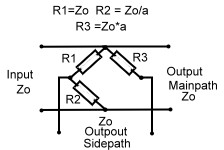S11=0; S22=0; S31=1/(1+a)

Fig.1 Wheatstone Bridge used as coupler

2.0 Wave Line and Twisted Wire Wave Line Combiners

Twisted wire wave line combiners and dividers are realized using a combination of  broadband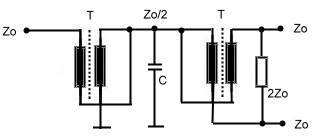wave line transformers. Due of the used technique they are restricted to relatively low frequencies, but they have the advantage to be very broad banded and very small in size. It is therefore sometimes desirable to realize such a combiner for high frequencies up to 1 GHz. This becomes clear, if one compares the size of an 3 dB strip line coupler having a dimension at 1 GHz of  7*7 cm, with the 0.5 cm square of a twisted wire combiner.

Fig.1 Twisted wire wave line transformer divider/combiner

A standard twisted wire wave line combiner is shown at Fig1.  Two equal wave line transformers are connected in series. The input transformer matches  the power dividing  output transformer. This arrangement may  be used as combiner or divider. The capacitor compensates the stray inductance’s at broadband applications. This circuit obviously is a three port. Using a simple 1:1 normal transformer realizes a four port device, Fig.2. Here, the transformer will limit the bandwith. A better RF- solution is it, to connect wave lines or twisted wire wave lines in a series- parallel circuitry, Fig.3 .The wave lines at Fig.3 may be replaced by twisted wire transformers, but if the Impedance of the twisted wire is not equal to Zo, phase deviations up to 25 degree will occur. There are many possibilities to build wave line combiners and dividers in a similar manner.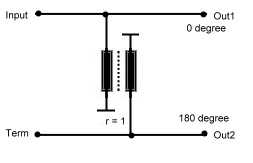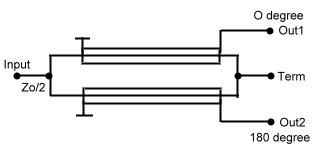Fig.2 Simple 4 port coupler            Fig.3 Paralleled Wave line coupler

One classical circuit at low frequencies is the Sontheimer coupler US Patent 3426298 of 1969. Fig.2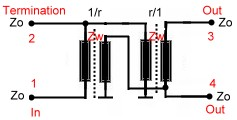Fig.2 Sontheimer Coupler

Practical Data’s of Twisted Wire and Wave Line combiners are:

• Signal phase shift : Phase = 0 or 180 degree.
• Working range range: f =1MHz to 1500 MHz.
• Bandwidth : B = 30%.
• Isolation :  up to 40 dB.
• Insertion loss S21 about 0.5dB.

Further readings wave line combiners :

R.E.Fisher, “ Broadband Twisted Wire Quadrature Hybrid, “ IEEE Transaction on Microwave Theory and Techniques.” Vol. MTT-21, May 1973

3.0 Line Hybrids

Hybrids in general are 90 degree combiner or quadrature couplers Fig.1 One of the two outputs has a phase difference of 90 degree referred to the input port. The fourth port is the isolated port, which must be terminated, but has normally no power on it. Line hybrids are  such 90 degree combiners and are very popular at frequencies in the UHF range.They divide the input power at port 1 to two output ports 2 and 4, having a loss of >3dB. Therefore they are called “ 3 dB couplers”. The two outputs have a phase shift of 90 degree. Port 3 must be terminated, to absorb reflected power in case of output mismatching; normally there is no power at this port.The input port matching therefore is relatively independed of the output matching.The mechanical construction is very simple.Two lambda/4  lines are coupled by means of a very good delectrica either in a cable or on a substrate.The mechanical length can be shortened if the lines are meander shaped The dimension of the coupler depends on the epsilon of the dielectric medium.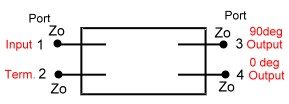In this hybrid, we have two impedance’s, the mutual impedance between the two lines Zm and the impedance’s from the lines to ground Zg. The coupler input impedance then is :

Zo = SQR(Zg Zm).Fig.2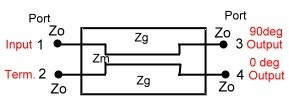Fig1. Basic Hybrid ^                           Fig.2 Line Hybrid

Practical Data’s of Line Hybrids are:

• Signal phase shift : Phase = 90 degree .
• Working range: 100 MHz to 2GHz.
• Bandwidth : B = 20% .
• Isolation = 30 dB.
• Insertion loss S21 >= 3dB .
• Input reflection S11>=20dB.

4.0 Brunch Line and Ring Couplers

Brunch line couplers are Hybrid couplers related to Fig.1 of 3.0 They have four lambda/4 branches , and are the classical microwave couplers. They are very easy to realize as PCB or micro strip on a substrate. But they have  the disatvandiges of low bandwidth, 90 degree phase between the two outputs, a loss of >= 3 dB and big dimensions at frequencies below 1 GHz. Fig.3 presents the mechanical drawing of the classical brunch coupler. Each branch has a length of lamdba/4 .Besides this arrangement other mechanical outlets are possible. If the input and output impedance’s are different , the brunch impedance will be:

Zo = SQR(Zin Zout /2):   Fig.2 shows a brunch coupler version having Lamba/8 matching stubs on the inputs .

A similar microwave coupler is the ring (or magic T coupler, this is a waveguide structure) and is shown at Fig.5. This hybrid may be used as combiner or divider if the inputs and outputs are exchanged. (green = divider, black is combiner.) The phase difference of the output is -180degree.

The hybrid transmission is a function of amplitude ratio and phase of the incoming signal.Therefore, to combine signals with an hybrid, they must be matched within a certain percentage in amplitude and phase.

Fig.1 Brunch line Coupler      Fig.2 Matched Brunch line Coupler   Fig.3 Ring Coupler (Magic T Hybrid )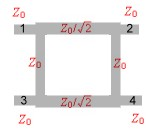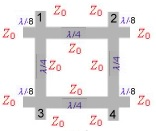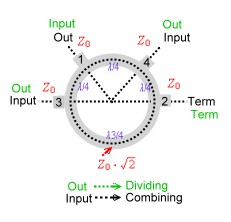Practical Data’s of brunch line and ring couplers are:

• Signal Phase Shift : Phase = 90 degree ( depends on the amplitude and phase balance of the input signal).
• Working range range: 1 GHz to 30 GHz.
• Bandwidth : B = 10 % ( may be higher using special matched arrangement).
• Isolation = 20 dB .
• Insertion loss >3dB.

5.0 Wilkinson Couplers

Wilkinson couplers or hybrids, are n- port couplers what means, signals on n-ports my either be added or divided. Indeed this coupler is not a hybrid ; therefore all outputs have the same phase of 90 degree related to the input. The basic idea, is a star network of transforming circuits balanced with lumped resistors Fig.1. The transforming networks can be either consisting of  L-C-Circiuts or many  wave line transformers. Fig. 2 shows a 50 Ohm two port divider and Fig.3 an practical strip line three port arrangement. For higher frequencies the balancing resistors may be a problem an must be induction free and hat sink.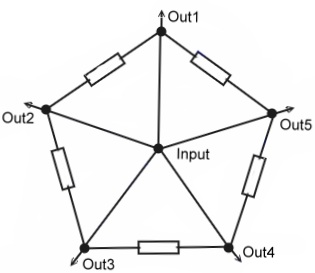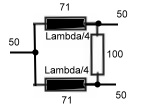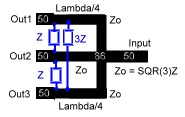Fig.1  Basic idea                        Fig.2 50Ohm divider        Fig.3 Three port divider